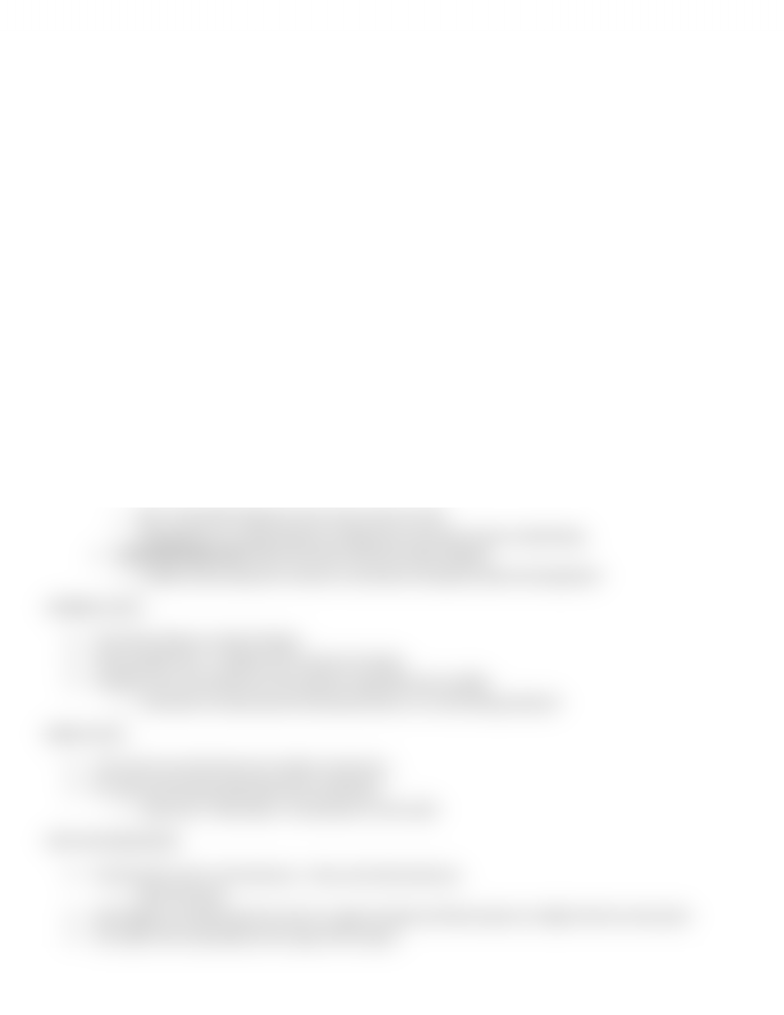Textbook Notes (280,000)
CA (160,000)
Queen's (3,000)
COMM (600)
Chapter 3

COMM 112 Chapter Notes - Chapter 3: Cost Driver, Fixed Cost, Variable Cost

Department
Commerce
Course Code
COMM 112
Professor
Teri Shearer
Chapter
3

This preview shows half of the first page. to view the full 2 pages of the document.BASICS OF COST BEHAVIOUR
Cost behaviour: the general tern for describing whether cost changes when the level of output changes
A cost that doesn’t change as total output changes is a FIXED COST
A cost that changes as output changes is a VARIABLE COST
Fixed and variable cost only exist when related to some output measure or drive
Cost driver measurement of the cause of the change in cost
o Predicting cost driver helps control costs better
The relevant range: the range of output over which the assumed cost relatoionship is valid for the normal
operations of a firm
o It limites the cost relationship to the range of operations that they firm normally expects to occur
FIXED COSTS:
Costs that in total are constant within the relevant range as the level of output increases/decreases
EX: a fleet of airplanes are fixed costs for the airlines becayse the cost doesn’t change as the number of flight
changes
EX: the rental cost per month is fixed it doesn’t change depending on how many sales are made
Higher output means that the fixed costs are spread over a wider range and therefore the cost is smaller per
unit
Two types of fixed costs:
1. Discretionary fixed costs: costs that can be changes or avoided relatively easily
o EX: advertising it depends on the decision by mangerment to purchase print, radio, or video
o BUT, it does NOT depend on how many units are sold
o Management can easily decide to change how much they invest in advertising
2. Committed fixed costs: fixed costs that cannot be easily changed
o Usually involve long-term contract or purchase of property, plant and equipment
VARIABLE COSTS:
Costs that change as output changes
Total variable costs = variable rate X amount of output
Variable costs can be fixed over the relevant range AKA semi-variable
o Economies of scale present this because there is so much being produced
MIXED COSTS:
Costs that have both fixed and variable components
EX: sales rep who gets paid salary plus commission
o Total costs = fixed salary + (commission X units sold)
STEP COST BEHAVIOUR
Cost functions aren't all continuous they can be discontinuous
o AKA semi-fixed
They display a constant level of cost for a range of output and then jumps to a higher level at some point
The width of the step defines the range of the output
You're Reading a Preview

Unlock to view full version